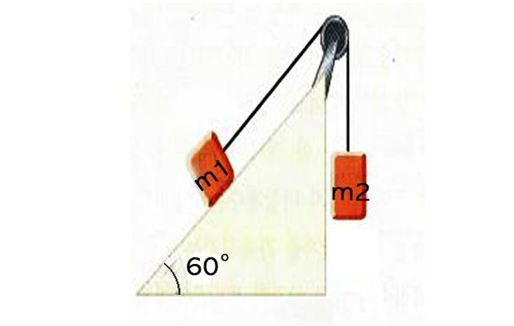# Friction between plane and objectIn the above diagram, a box with mass $m_{1} (= 8 \text{ kg}$) is on a slope with $60^{\circ}$ inclination. This box is connected with another box with mass $m_{2} (= 13 \text{ kg}$) by a wire on a frictionless pulley. What is the magnitude of the acceleration of the two boxes when the coefficient of kinetic friction between the first box $( 8 \text{ kg} )$ and the slope is $0.2?$

Gravitational acceleration is $g=10\text{ m/s}^{2}.$

×

Problem Loading...

Note Loading...

Set Loading...